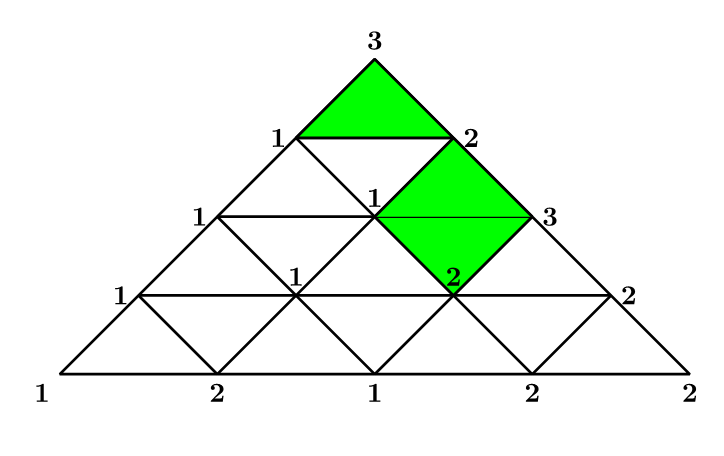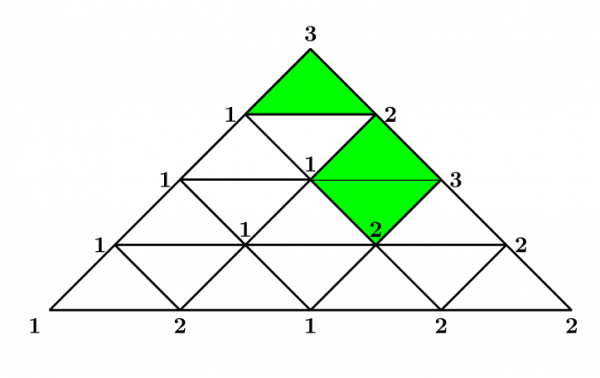HighlightsCutting Cakes and Splitting Rent – Combinatorics and Fair Division

MSRI - January 2019How do you divide a cake fairly among several people with different preferences?  How do you assign rooms and divide the rent among roommates so that no one envies the room and portion of rent paid by the other?  Both of these are questions of fair-division, and mathematicians have shown that answers can be found using a combinatorial result known as Sperner’s Lemma.

Sperner’s Lemma says the following:  Take a triangle $T$ and label the vertices 1, 2, 3.  Divide the triangle into smaller triangles as in the figure below.  Now label the vertices of the smaller triangle with the numbers 1, 2, 3, but obey the rule:  if a vertex lies on a side of the original triangle, it must be labelled with the same label as one of the endpoints of that edge (look at the right edge of the triangle – all three vertices there are labelled 2 or 3).  Sperner’s Lemma says that when you are finished there will be an odd number of the smaller triangles whose vertices are labelled 1, 2, 3 (in the figure there are three).  In particular there will be at least one such.  One can give proofs of the lemma that are constructive and these allow one to find solutions to fair-division problems.This result has several generalizations, many of which were topics studied during the Geometric and Topological Combinatorics program at MSRI in Fall 2017.  Previously, Florian Frick, Frédéric Meunier and Kelsey Houston-Edwards proved a result that implies the “Secretive Roommate Theorem”:  there exists a division of rent among roommates even if one roommate is “secretive” or is not present in the room when the division is decided.  Generalizations concerning labelled triangulations of higher dimensional simplices are also known.  During the program, Frédéric Meunier and Francis Su proved a multilabelled version of Sperner’s Lemma, which implies many of the above results as well as yielding a “Survivor-Style” Cake-Cutting Theorem:  For any $N$ people, there exists a division of a cake into $N-1$ pieces such that, no matter which person decides to leave, the $N-1$ remaining people can select pieces in an envy-free way.  Sperner’s Lemma and its generalization have applications, from matching medical students with hospitals for their residency to theoretical questions in topology.  These new tools will yield further new results.

The Fall 2017 program at MSRI yielded many other new results and served as a catalyst for Geometric and Topological Combinatorics, a fast expanding and active field with many applications to mathematical economics, optimization, and social choice theory as well as to traditional mathematical fields such as algebraic geometry, discrete geometry and topology.